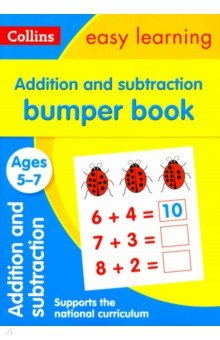Серия: "Collins Easy Learning" An engaging bumper Addition and Subtraction activity book to really help boost your child's progress at every stage of their learning! Including helpful questions and answers, this Maths book provides reassurance whilst supporting your child's learning at home. Combining useful Maths practice with engaging, colourful illustrations, this Addition and Subtraction bumper practice book helps to boost your child's confidence and develop good learning habits for life. Each fun activity is designed to give your child a real sense of achievement. Издательство: "Harper Collins UK" (2018) Категории: ISBN: 978-0-00-827546-4

Другие книги схожей тематики:

АвторКнигаОписаниеГодЦенаТип книги
Addition and Subtraction Bumper BookAn engaging bumper Addition and Subtraction activity book to really help boost your child`s progress at every stage of their learning! Including helpful questions and answers, this Maths book… — HarperCollins Publishers, Easy Learning Подробнее...2018
1239бумажная книга

Look at other dictionaries:

• Addition-subtraction chain — An addition subtraction chain, a generalization of addition chains to include subtraction, is a sequence a 0, a 1, a 2, a 3, ... that satisfies:a 0 = 1, ,: ext{for }k > 0, a k = a i pm a j ext{ for some }0 leq i,j < k.An addition subtraction… …   Wikipedia

• Subtraction — 5 − 2 = 3 (verbally, five minus two equals three ) …   Wikipedia

• Addition-chain exponentiation — In mathematics and computer science, optimal addition chain exponentiation is a method of exponentiation by positive integer powers that requires a minimal number of multiplications. It works by creating a minimal length addition chain that… …   Wikipedia

• subtraction — Compound Com pound, a. [OE. compouned, p. p. of compounen. See {Compound}, v. t.] Composed of two or more elements, ingredients, parts; produced by the union of several ingredients, parts, or things; composite; as, a compound word. [1913 Webster] …   The Collaborative International Dictionary of English

• Addition chain — In mathematics, an addition chain is a sequence a 0, a 1, a 2, a 3, ... that satisfies: a 0 = 1, and :for each k >0: a k = a i + a j for some i , j < k .As an example: 1, 2, 3, 6, 12, 24, 30, 31 is an addition chain for 31, of length 7, since:2 …   Wikipedia

• Addition — is the mathematical process of putting things together. The plus sign + means that two numbers are added together. For example, in the picture on the right, there are 3 + 2 apples meaning three apples and two other apples which is the same as… …   Wikipedia

• addition — [n1] process of conjoining, adding accession, adding, adjoining, affixing, annexation, attachment, augmentation, enlargement, extension, inclusion, increasing; concepts 236,245 Ant. decrease, deduction, lessening, loss, reduction, shrinkage,… …   New thesaurus

• Subtraction without borrowing — A commonly known subtraction method is the borrowing method [Only a brief, informal discussion is included here until a formal entry of dynamic subtraction is provided.] . To perform a b using this method, b , the subtrahend, is written below a …   Wikipedia

• addition — noun 1 sth that is added; process of adding sth ADJECTIVE ▪ useful, valuable, welcome, worthy ▪ important, major, notable, significant ▪ …   Collocations dictionary

• addition — ad|di|tion W1S3 [əˈdıʃən] n 1.) in addition used to add another piece of information to what you have just said ▪ The company provides cheap Internet access. In addition, it makes shareware freely available. in addition to sth ▪ In addition to… …   Dictionary of contemporary English